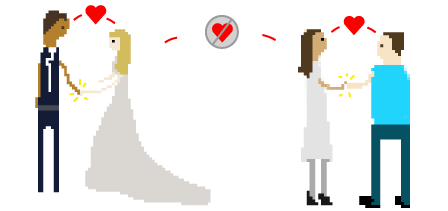# On the fifth day of Christmas, Calvin gave to me

Probability Level 25 golden rings

At the registry of marriage, there were 5 couples (total of 10 people) that were waiting to get married. If we pick any two people out of these 10, what is the probability that they are a couple?

1) $\frac{1}{5}$
2) $\frac{ 1}{9}$
3) $\frac{1}{10}$
4) $\frac{1}{45}$

×